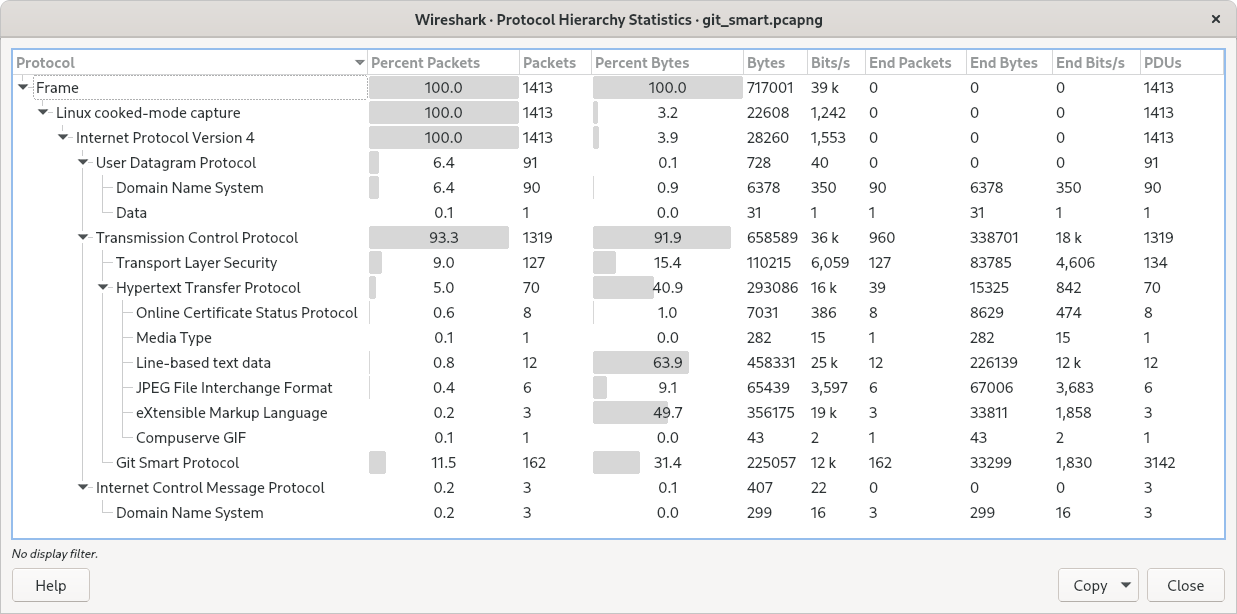## 8.4. The “Protocol Hierarchy” Window

The protocol hierarchy of the captured packets.

Figure 8.3. The “Protocol Hierarchy” WindowThis is a tree of all the protocols in the capture. Each row contains the statistical values of one protocol. Two of the columns (Percent Packets and Percent Bytes) serve double duty as bar graphs. If a display filter is set it will be shown at the bottom.

The button will let you copy the window contents as CSV or YAML.

Protocol hierarchy columns

Protocol
This protocol’s name.
Percent Packets
The percentage of protocol packets relative to all packets in the capture.
Packets
The total number of packets that contain this protocol.
Percent Bytes
The percentage of protocol bytes relative to the total bytes in the capture.
Bytes
The total number of bytes of this protocol.
Bits/s
The bandwidth of this protocol relative to the capture time.
End Packets
The absolute number of packets of this protocol where it was the highest protocol in the stack (last dissected).
End Bytes
The absolute number of bytes of this protocol where it was the highest protocol in the stack (last dissected).
End Bits/s
The bandwidth of this protocol relative to the capture time where was the highest protocol in the stack (last dissected).
PDUs
The total number of PDUs of this protocol.

Packets usually contain multiple protocols. As a result, more than one protocol will be counted for each packet. Example: In the screenshot 100% of packets are IP and 99.3% are TCP (which is together much more than 100%).

Protocol layers can consist of packets that won’t contain any higher layer protocol, so the sum of all higher layer packets may not sum to the protocol’s packet count. This can be caused by segments and fragments reassembled in other frames, TCP protocol overhead, and other undissected data. Example: In the screenshot 99.3% of the packets are TCP but the sum of the subprotocols (TLS, HTTP, Git, etc.) is much less.

A single packet can contain the same protocol more than once. In this case, the entry in the `PDUs` column will be greater than that of `Packets`. Example: In the screenshot there are many more TLS and Git PDUs than there are packets.